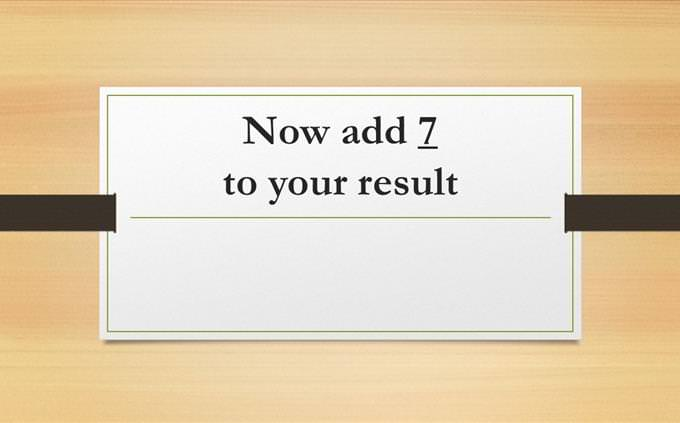# Can You Do Math and Remember as Well?

The following quiz is a really fun challenge that requires two important capabilities: The first is to do math in your head, the second is your short term memory. We will show you a number, then you will be asked in each slide to perform a simple math operation with that number (add, subtract, multiply or divide) while choosing the right answers until you get to the correct final one! In the end, if you fail, you can find out where you went wrong and try again. Can your memory hold the math? Let's find out!Hold the following sequence of math operations in your head, and select the number you are supposed to reach each time.
8
6
1615
23
1320
28
1810
9
147
6
1142
36
66126
144
108143
153
161202
212
220101
106
9886
84
83172
182
162168
170
167You Took a False Step
Somewhere in your calculations you went wrong, and so did not get all answers correct. Below you will see where your answers started being wrong, to see where you lost the thread. You can try again starting from that point or restart it to see if your memory can hold better this time!